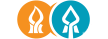In collaboration with Ministry of Science and Technology of Israel

### Search problems in the domain of multiplication: Case study on anomaly detection using markov chainsY Mirsky, A Cohen, R Stern, A Felner, L Rokack, Y Elovici, B Shapira

Eighth Annual Symposium on Combinatorial Search, 2015

Most work in heuristic search focused on path finding problems
in which the cost of a path in the state space is the sum
of its edges’ weights. This paper addresses a different class
of path finding problems in which the cost of a path is the
product of its weights. We present reductions from different
classes of multiplicative path finding problems to suitable
classes of additive path finding problems. As a case study,
we consider the problem of finding least and most probable
paths in a Markov Chain, where path cost corresponds to the
probability of traversing it. The importance of this problem
is demonstrated in an anomaly detection application for cyberspace
security. Three novel anomaly detection metrics for
Markov Chains are presented, where computing these metrics
require finding least and most probable paths. The underlying
Markov Chain is dynamically changing, and so fast methods
for computing least and most probable paths are needed. We
propose such methods based on the proposed reductions and
using heuristic search algorithms.